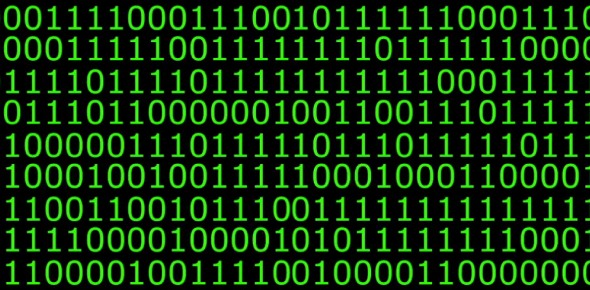# Binary Form Quiz Assessment

8 Questions | Attempts: 363
ShareSettingsThis quiz is intended to test you on your knowledge of binary form, based on the content from Clarion's PODs Projects.

• 1.
A ______ form is musical form that consists of two approximately equivalent sections, although they may be of an equal length.
• 2.
Which of the following diagrams best represents the most basic variety of binary form?
• A.

| A |

• B.

| A | B |

• C.

| A | B | C |

• D.

| A | B | C | D |

• 3.
Which diagram best represents "two-reprise" binary?
• A.

| A | B |

• B.

||: A :|| B |

• C.

| A ||: B :||

• D.

||: A :||: B :||

• 4.
True or False:Continuous binary ends the first section of a form on the tonic triad.
• A.

True

• B.

False

• 5.
What chord does the first half of a sectional binary example end on? (in a major key)(answer with a roman numeral)
• 6.
What kind of music is binary form most often found in?
• A.

Renaissance

• B.

Baroque

• C.

Classical

• D.

Romantic

• 7.
True or False:Just because a song has an introduction, does not mean it is in binary form.
• A.

True

• B.

False

• 8.
True or False:If the end of the first section of a binary example modulates, this is known as continuous binary.
• A.

True

• B.

False

## Related TopicsBack to top
×

Wait!
Here's an interesting quiz for you.